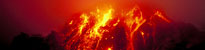It is more efficient to use a queue for reading and saving samples because your computer may not be able to process these chunks of information in one go. Queuing allows the samples to always be read, independently of saving them, so they are not slowed by the saving process.

Note there are two different types of queue; first in first off (FIFO), and last in first off (LIFO). These are as you would think, you either take off the last or the first. The default for Python is FIFO, which we will use.

Also we will use Threading, as this is essential for our queue Threading allows multitasking. We use this to run multiple 'threads' (tasks), one for reading and one for saving, so that they run at their own speeds.

## Programming

To start we need to import the correct libraries. With the previous two we had, NumPy and ObsPy, we now need two more, queue and threading:

import numpy
from obspy.core import Trace,Stream,UTCDateTime
import Queue

We need to declare a few variables: block length, this is the same as datapoints. Block id, this is to remember the names uses to write files, and this must be global (so it can be reused when we restart the function to save data). And of course we must declare the queue.

#this is how after how many samples a block is saved
block_length=128
#this is needed for saving in mseed so must be passed globably
global starttime
#define the queue from the function queue in the library queue
q = Queue.Queue()

Next we have the function for reading data. This is not threaded, it is a normal function. This is a just a function of the read data loop in the last program, including a start time. And instead of saving the values to an array, it just queues them. See how it queues the value; using a function of the queue. This is the same for all queue operations, as we again see soon. For more information see Python queue operations.

starttime=UTCDateTime()
for x in range (block_length):
#loop continues for block length
#'timenow' not essential at the moment and isn't stored
timenow=UTCDateTime()
print sample,timenow
queue.put(sample)

Next is the thread below. So this will run at an independent rate to the last function. It must have an infinite loop, otherwise the thread will end. It begins checking the size of our queue, if it has enough for a block (a file of 128 values), by using a queue function giving its length.

Like the last program it saves the data into a NumPy array, by a loop going through queue getting 128 (the block length) values. Note the queue functions, get() just returns the value at the front, and this must be checked as done, with task_done().

As before we get all the stats for the header, and stream & trace these. Then we can save this. As you may want to run this for more than one block, it will name it with 'MSEED' and a number, incrementing for each block, and in a folder called mseed — you should create this now, before running the program.

def save_data():
#iterator for writing files
global block_id
block_id=0
while True:
if queue.qsize() >= block_length:
data=numpy.zeros([block_length],dtype=numpy.int16)
for x in range (block_length):
sample = queue.get()
data[x]=sample
stats = {'network': 'UK', 'station': 'PHYS', 'location': '00', 'channel': 'BHZ', 'npts': block_length, 'sampling_rate': 20, 'mseed': {'dataquality': 'D'},'starttime': starttime}
stream.write('mseed/MSEED' + str(block_id) + '.mseed',format='MSEED',encoding='INT16',reclen=512)
block_id += 1

Now we just need to set-up the function as the thread, then call the read data function. I have called it so it gets five block worths of values, but you can change this.

for x in range(5):

When this is all done the program should at the start save your data, and won't stop ever, as the thread is continuous, so hit ctrl-z (undo) when it has saved everything.

## Next step... jitter and sampling rate

The next step to advance our program is to include another measurement, the jitter.

This is a useful measurement as it records how well our program and ADC are functioning.— see Jitter and sampling rateEarthquakes: how, why, where and when?
School seismology
FootballQuake
MarsQuake
News, teaching and museums